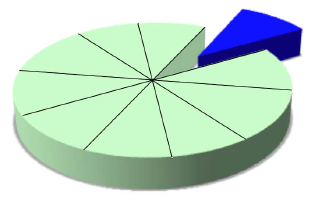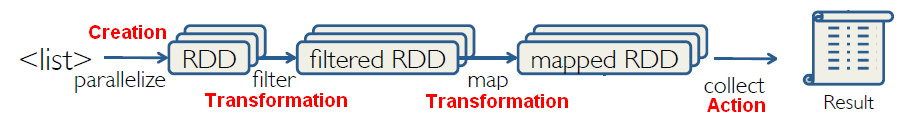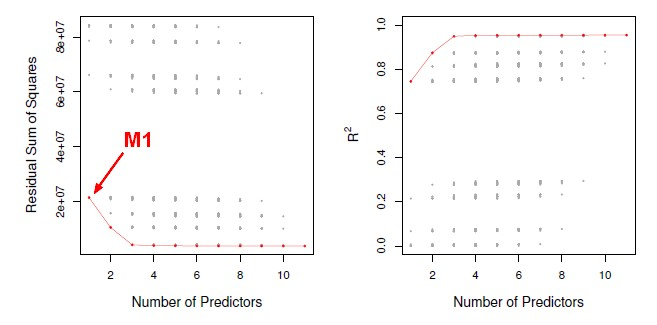# Statistics - Resampling through Random Percentage Split

Percentage Split (Fixed or Holdout) is a re-sampling method that leave out random N% of the original data.

For example, you might select:

The algorithm is trained against the trained data and the accuracy is calculated on the test data set.

## Standard Deviation in Validation

When percentage split with a random method is repeated for validation, there is a good chance of overlap between the different test sets. The algorithm has already (learn|see) them. With cross-validation, this overlap doesn't occur. This is why the standard deviation estimate tends to be smaller for percentage split than for cross-validation.

Recommended Pages(Statistics|Data Mining) - (K-Fold) Cross-validation (rotation estimation)

Cross-validation, sometimes called rotation estimation is a resampling validation technique for assessing how the results of a statistical analysis will generalize to an independent new data set. This...Data Mining - (Test|Expected|Generalization) Error

Test error is the prediction error that we incur on new data. The test error is actually how well we'll do on future data the model hasn't seen. The test error is the average error that results from using...Model Building - ReSampling Validation

Resampling method are a class of methods that estimate the test error by holding out a subset of the training set from the fitting process, and then applying the statistical learning method to those held...R - Feature Selection - Model selection with Direct validation (Validation Set or Cross validation)

Feature selection through model generation and selection through a direct approach with : validation set and cross validation in R We are picking a subset of the observations and put them...Spark - (Random) Split

randomSplit randomly splits a RDD with the provided weights. where: weights – weights for splits, will be normalized...Statistics - Model Selection

Model selection is the task of selecting a statistical model from a set of candidate models through the use of criteria's Dimension reduction procedures generates and returns a sequence of possible...